#### TheAreaMethod

###### back to index

A circle of radius 2 is centered at $A$. An equilateral triangle with side 4 has a vertex at $A$. What is the difference between the area of the region that lies inside the circle but outside the triangle and the area of the region that lies inside the triangle but outside the circle?

Three congruent isosceles triangles are constructed with their bases on the sides of an equilateral triangle of side length $1$. The sum of the areas of the three isosceles triangles is the same as the area of the equilateral triangle. What is the length of one of the two congruent sides of one of the isosceles triangles?

A rectangle of perimeter 22 cm is inscribed in a circle of area $16\pi$ $cm^2$. What is the area of the rectangle? Express your answer as a decimal to the nearest tenth.

Convex quadrilateral $ABCD$ has $AB=3$, $BC=4$, $CD=13$, $AD=12$, and $\angle ABC=90^{\circ}$, as shown. What is the area of the quadrilateral?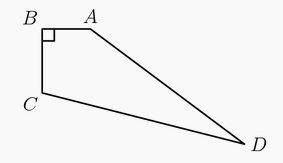$ABCD$ is a square of side length $\sqrt{3} + 1$. Point $P$ is on $\overline{AC}$ such that $AP = \sqrt{2}$. The square region bounded by $ABCD$ is rotated $90^{\circ}$ counterclockwise with center $P$, sweeping out a region whose area is $\frac{1}{c} (a \pi + b)$, where $a$, $b$, and $c$ are positive integers and $\text{gcd}(a,b,c) = 1$. What is $a + b + c$?

Equilateral triangle ABC with side-length 12 cm is inscribed in a circle. What is the area of the largest equilateral triangle that can be drawn with two vertices on segment AB and the third vertex on minor arc AB of the circle? Express your answer in simplest radical form.

A circle of radius 5 is inscribed in a rectangle as shown. The ratio of the length of the rectangle to its width is 2:1. What is the area of the rectangle?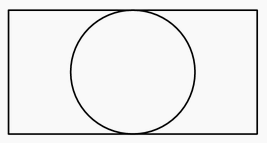A trapezoid has side lengths 3, 5, 7, and 11. The sums of all the possible areas of the trapezoid can be written in the form of $r_1\sqrt{n_1}+r_2\sqrt{n_2}+r_3$, where $r_1$, $r_2$, and $r_3$ are rational numbers and $n_1$ and $n_2$ are positive integers not divisible by the square of any prime. What is the greatest integer less than or equal to $r_1+r_2+r_3+n_1+n_2$?

Circles $A, B,$ and $C$ each have radius 1. Circles $A$ and $B$ share one point of tangency. Circle $C$ has a point of tangency with the midpoint of $\overline{AB}.$ What is the area inside circle $C$ but outside circle $A$ and circle $B?$

Circles with radii $1$, $2$, and $3$ are mutually externally tangent. What is the area of the triangle determined by the points of tangency?

Rhombus $ABCD$ has side length $2$ and $\angle B = 120$\u00b0. Region $R$ consists of all points inside the rhombus that are closer to vertex $B$ than any of the other three vertices. What is the area of $R$?

A big $L$ is formed as shown. What is its area?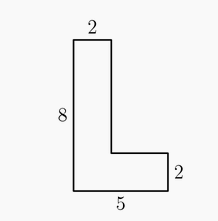Andrea inscribed a circle inside a regular pentagon, circumscribed a circle around the pentagon, and calculated the area of the region between the two circles. Bethany did the same with a regular heptagon (7 sides). The areas of the two regions were $A$ and $B$, respectively. Each polygon had a side length of $2$. Which of the following is true?

A rectangular yard contains two flower beds in the shape of congruent isosceles right triangles. The remainder of the yard has a trapezoidal shape, as shown. The parallel sides of the trapezoid have lengths $15$ and $25$ meters. What fraction of the yard is occupied by the flower beds?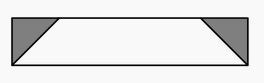Five unit squares are arranged in the coordinate plane as shown, with the lower left corner at the origin. The slanted line, extending from $(a,0)$ to $(3,3)$, divides the entire region into two regions of equal area. What is $a$?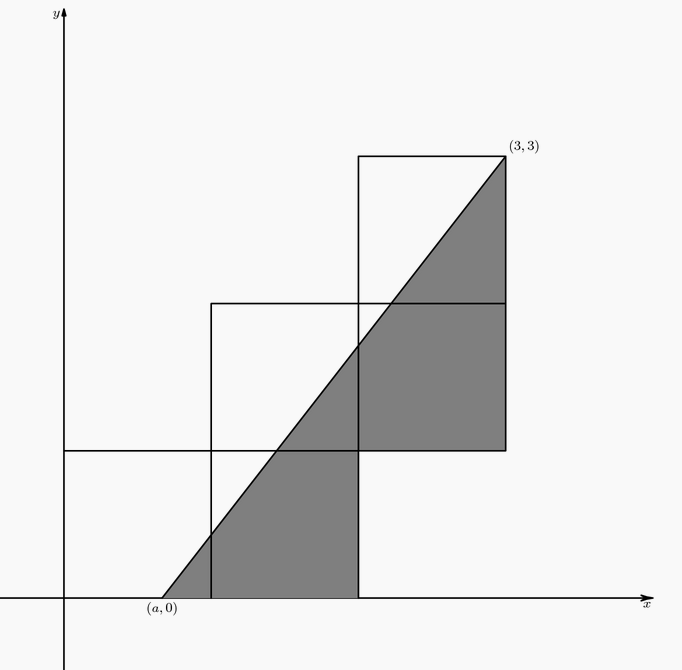What is the area of the region defined by the inequality $|3x-18|+|2y+7|\le3$?

On circle $O$, points $C$ and $D$ are on the same side of diameter $\overline{AB}$, $\angle AOC = 30^\circ$, and $\angle DOB = 45^\circ$. What is the ratio of the area of the smaller sector $COD$ to the area of the circle?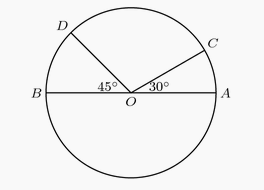Vertex $E$ of equilateral $\triangle{ABE}$ is in the interior of unit square $ABCD$. Let $R$ be the region consisting of all points inside $ABCD$ and outside $\triangle{ABE}$ whose distance from $AD$ is between $\frac{1}{3}$ and $\frac{2}{3}$. What is the area of $R$?

Let $ABCD$ be a trapezoid with $AB||CD, AB=11, BC=5, CD=19,$ and $DA=7$. Bisectors of $\angle A$ and $\angle D$ meet at $P$, and bisectors of $\angle B$ and $\angle C$ meet at $Q$. What is the area of hexagon $ABQCDP$?

Points $A,B,C,D$ and $E$ are located in 3-dimensional space with $AB=BC=CD=DE=EA=2$ and $\angle ABC=\angle CDE=\angle DEA=90^o$. The plane of $\triangle ABC$ is parallel to $\overline{DE}$. What is the area of $\triangle BDE$?

The vertices of a $3-4-5$ right triangle are the centers of three mutually externally tangent circles, as shown. What is the sum of the areas of the three circles?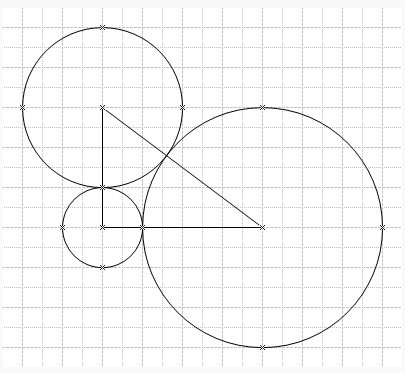Rhombus $ABCD$ is similar to rhombus $BFDE$. The area of rhombus $ABCD$ is 24, and $\angle BAD = 60^\circ$. What is the area of rhombus $BFDE$?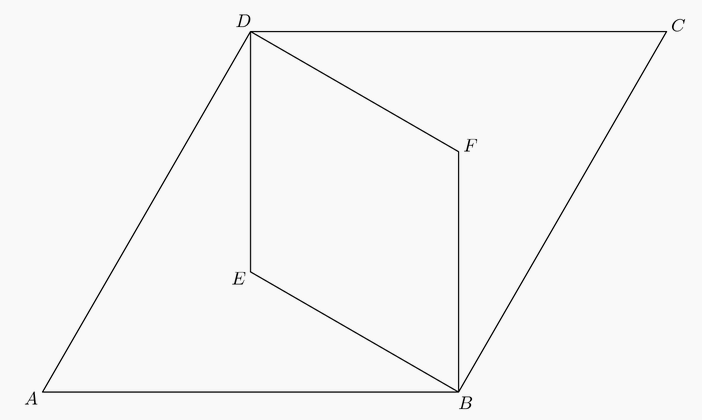Circles with centers $O$ and $P$ have radii 2 and 4, respectively, and are externally tangent. Points $A$ and $B$ are on the circle centered at $O$, and points $C$ and $D$ are on the circle centered at $P$, such that $\overline{AD}$ and $\overline{BC}$ are common external tangents to the circles. What is the area of hexagon $AOBCPD$?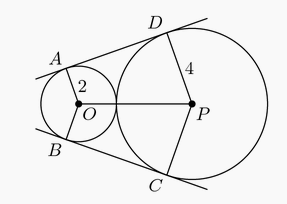A rectangle with diagonal length $x$ is twice as long as it is wide. What is the area of the rectangle?

Square $EFGH$ is inside the square $ABCD$ so that each side of $EFGH$ can be extended to pass through a vertex of $ABCD$. Square $ABCD$ has side length $\sqrt {50}$ and $BE = 1$. What is the area of the inner square $EFGH$?